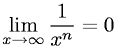Equations > Calculus > Limits > Limit of One over X to the nth Power

### Limit of One over X to the nth PowerLatex Code:

MathML Code:

 $\underset{x\to \infty }{lim}\frac1{x}^{n}=0$

MathType 5.0: